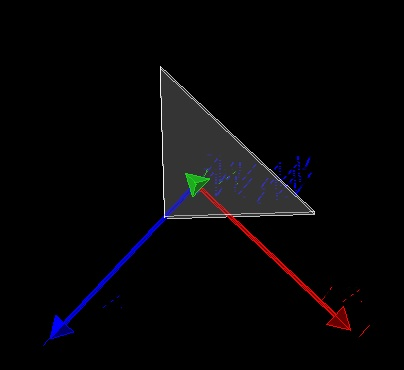# How to define a triangular prism volume, help!someone can tell me how to defien a triangular prism volume

To find the volume of a right prism, just multiply the area of the base by the height.
Below is a link where you can find the expression for a triangular prism:

does this post help?

Thank you for your prompt reply,i wil try to define a triangular prism Using the above methods

You can also create a triangular prism using G4Trd, for example:

`G4Trd("Prism", 100*cm, 0*cm, 200*cm, 200*cm, 80*cm)`

using the above method can only creat a Acute triangular prism , but i want to define a rectangular triangular prism, can you have any else method to creat it ?

Using G4Trd you can create a triangular prism with any isosceles triangle at its base, including a right-angled one, for example

`G4Trd("Prism", 100*cm, 0*cm, 200*cm, 200*cm, 50*cm)`A triangular prism with arbitrary triangle at its base can be created using G4ExtrudedSolid.
An example:

``````std::vector<G4TwoVector> triangle(3);
triangle = G4TwoVector(-50*cm, -50*cm);
triangle = G4TwoVector(-30*cm, 120*cm);
triangle = G4TwoVector( 70*cm, -80*cm);
G4ExtrudedSolid* solidPrism = new G4ExtrudedSolid("Prism", triangle, 200*cm,
G4TwoVector(0,0), 1., G4TwoVector(0,0), 1.);
``````

Thank you for your help. I have another question. In this case, how to determine the center of this triangular prism

Do you mean the center of gravity? Тo find the center of gravity, you need to divide the sum of all vertices by six.

Please note, that the axes are oriented differently in the case of G4Trd and the case of G4ExtrudedSolid. It means that to place the prism in the world volume you should apply different transformations.

Thank you, Sir, but I don’t know if moving the center of gravity can move this volume. I want to put this triangular prism in a correct position, so I need to know the center that defines this volume

To place the prism in the required position you should specify a transformation. I would recommend to use G4Transform3D for that. With this class you can subdivide the movement of the prism in a sequence of simple steps and find the needed transformation as a product of G4Transform3D objects.

This topic was automatically closed 7 days after the last reply. New replies are no longer allowed.